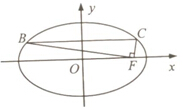• 在?ABC中,角A,B,C所对的边分别为a,b,c,∠ABC=120o,∠ABC的平分线交AC于点D,且BD=1,则4a+c的最小值

在?ABC中，角A,B,C所对的边分别为a,b,c，∠ABC=120o，∠ABC的平分线交AC于点D，且BD=1，则4a+c的最小值为_______．

• (本小题满分14分) 已知a,β为锐角,tana=4/3,cos(a+β)=-√5/5.(1)求cos2a的值; (2)求tan(a-β)的值

（本小题满分14分） 已知a,β为锐角，tana=4/3，cos(a+β)=-√5/5．

（1）求cos2a的值； （2）求tan(a-β)的值．

• 若函数f(x)=2x^3-ax^2+1(a∈R)在(0,+∞)内有且只有一个零点,则f(x)在[-1,1]上的最大值与最小值的和

若函数f(x)=2x^3-ax^2+1(a∈R)在(0,+∞)内有且只有一个零点，则f(x)在[-1,1]上的最大值与最小值的和为_______．该问题答案仅对会员开放，欢迎开通会员

1个月
¥39.8
1.28元/天
3个月
¥49.8
0.54元/天
1周
¥29.8
4.26元/天微信支付支付宝支付### 请使用微信扫码支付(元)